# The volume 6

The volume of a sphere is given by the formula 4/3 πr3 (or 4/3 *pi *r3) . The value of pi is approximately equal to 3.14. What is the volume of a sphere whose radius is 4 cm? (Round of the answer to the nearest hundredths. Type the value without the unit)

V =  268.08 cm3

### Step-by-step explanation: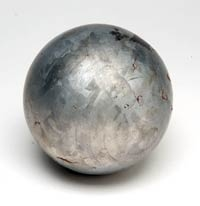Did you find an error or inaccuracy? Feel free to write us. Thank you!Tips to related online calculators
Tip: Our volume units converter will help you with the conversion of volume units.
Do you want to round the number?

#### You need to know the following knowledge to solve this word math problem:

We encourage you to watch this tutorial video on this math problem:

## Related math problems and questions:

• Video gameNicole is playing a video game where each round lasts 7/12 of an hour. She has scheduled 3 3/4 hours to play the game. How many rounds can Nicole play?
• Unknown metal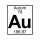The prism made of unknown metal has dimensions of 3 cm by 3 cm by 5 cm and a weight of 121 g. What metal is it? Write its chemical symbol.
• Chester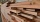Chester has a piece of wood measuring 1 2/3 cm. He needs to cut it to pieces measuring 3/4 cm long. How many pieces of wood did Chester cut?
• Food weightStacie is a resident at the medical facility where you work. You are asked to chart the amount of solid food that she consumes. For the noon meal, today she ate 1/2 of a 3-ounce serving of meatloaf, 3/4 of her 3-ounce serving of mashed potatoes, and 1/3 o
• SimilarityABC is a triangle wherein a = 4 cm, b = 6 cm, c = 8 cm. Is it similar to the triangle DEF: d = 3 cm, e = 4.5 cm, f = 6 cm? If so, determine the ratio of similarity.
• The granite ballWhat is the weight of a granite ball with a radius of 60 cm, if 1 m3 of granite weighs 2.7 tons?
• FlourKim needs 3/4 cup of flour to make 12 cookies. How much flour would she need to make 60 cookies?
• Body diagonalCalculate the volume of a cuboid whose body diagonal u is equal to 6.1 cm. Rectangular base has dimensions of 3.2 cm and 2.4 cm
• Plasticine ballPlasticine balls have radius r1=85 cm, r2=60 mm, r3=59 cm, r4=86 cm, r5=20 cm, r6=76 mm, r7=81 mm, r8=25 mm, r9=19 mm, r10=14 cm. For these balls
• Water levelThe glass container has a cuboid shape with dimensions of the bottom 24 cm and 12 cm. The height of the water is 26 cm. Calculate the body's volume, which is sunk into the water if the water level rise by 3 cm.
• Three rectangles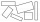Some wire is used to make 3 rectangles: A, B, and C. Rectangle B's dimensions are 3/5 cm larger than Rectangle A's dimensions, and Rectangle C's dimensions are again 3/5 cm larger than Rectangle B's dimensions. Rectangle A is 2 cm by 3 1/5 cm. What is the
• I need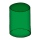I need to calculate the height of the cylinder. I have a given that the radius is 6 cm and the volume is 282.6 cm3. What is the formula for this?
• Median or middle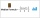The number of hours of television watched per day by a sample of 28 people is given below: 4, 1, 5, 5, 2, 5, 4, 4, 2, 3, 6, 8, 3, 5, 2, 0, 3, 5, 9, 4, 5, 2, 1, 3, 4, 7, 2, 9 What is the median value?
• Hollow sphere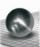The volume of the hollow ball is 3432 cm3. What is its internal radius when the wall thickness is 3 cm?
• Colored paperCan a 4 cm, 3 cm and 2 cm block be glued with a colored quarter of paper measuring 7 cm and 8 cm?
• Volume of the coneFind the volume of the cone with the base radius r and the height v. a) r = 6 cm, v = 8 cm b) r = 0,9 m, v = 2,3 m c) r = 1,4 dm, v = 30 dm
• Rotating coneCalculate volume of a rotating cone with base radius r=12 cm and height h=7 cm.